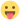## Binomial distribution (distrACTION)

Discuss statistics related things

## Binomial distribution (distrACTION)

Hello,
DistrACTION sometimes provides an incorrect answer. Here is an example.
Suppose that X is a random variable following a binomial law of parameters n = 12 and p = 0.3. Calculate P(X >= 6.5). According to Jamovi, P(X >= 6.5) = 0.118 (see DistrACTION_example_01.png), while P(X >= 7) = 0.039 (see DistrACTION_example_02.png). Now we should get the same result ! Isn't it a bug?
Hoping that my message is clear and that you will be able to answer me, I send you my cordial greetings.

JantonieP(X >= 7)
DistrACTION_example_02.png (86.76 KiB) Viewed 1319 timesP(X >= 6.5)
DistrACTION_example_01.png (92.52 KiB) Viewed 1323 times
jantonie

Posts: 22
Joined: Tue Oct 17, 2017 11:58 am

## Re: Binomial distribution (distrACTION)

hi jantonie,

you could try creating an issue over at the destrACTION project's github repo:

https://github.com/Mrihs/distrACTION

if you've got some R skills, you may even be able to identify the issue yourself.

if you don't get a response from the developers for a couple of weeks (it's the time of year where things slow down a bit), then come back to me, and i'll see what we can do.

cheers

jonathonjonathon

Posts: 1729
Joined: Fri Jan 27, 2017 10:04 am

## Re: Binomial distribution (distrACTION)

jantonie wrote:Now we should get the same result ! Isn't it a bug?
Hoping that my message is clear and that you will be able to answer me, I send you my cordial greetings.

Hi, @jantonie.
This is not a bug, but it is the one's complement probability of the r pbinom() function used in DistrACTION, which takes only the integer of the number of successes.
For your example its use would be:
P(X>=x) = 1 - pbinom (x-1, 12, 0.3)
Take a look at the screenshot that I am attaching, with a little script with Rj it will be more useful.Pbinom.PNG (70.95 KiB) Viewed 1300 times

Cheers,
MaurizioMAgojam

Posts: 155
Joined: Thu Jun 08, 2017 2:33 pm
Location: Parma (Italy)

## Re: Binomial distribution (distrACTION)

Hello,

In my opinion, this is a bug. Even if the modalities of a binomial variable are discrete, it should be possible to correctly calculate the value of the distribution function for any real number. Here's how the bug could be fixed.
I will try to make a proposal in github, but here is already the general idea :

For P(X <= x1):
DistributionResult <- pbinom(x1, size, prob)

For P(X >= x1):
DistributionResult <- 1 - pbinom(x1, size, prob) + dbinom(x1, size, prob)

For P(x1 <= X <= x2):
DistributionResult <- pbinom(x2, size, prob) - pbinom(x1, size, prob) + dbinom(x1, size, prob)

Best regards

Jantonie

P. S.: In DistrACTION, it would also be nice to be able to change the accuracy of the results displayed...
jantonie

Posts: 22
Joined: Tue Oct 17, 2017 11:58 am

## Re: Binomial distribution (distrACTION)

Hello,

Another small remark concerning the example that MAgojam proposes. We see that according to Jamovi P(X ≥ 6.5) = 0.118. What we also see on the figure, is that P(X ≥ 6.5) = P(X=7) + P(X=8) + ... + P(X=12) (the probability sought is represented by orange bars). According to R,

> sum(dbinom(7:12, 12, 0.3))
 0.03860084

Best regards

Jantonie
jantonie

Posts: 22
Joined: Tue Oct 17, 2017 11:58 am

## Re: Binomial distribution (distrACTION)

Hi, Jantonie.
I do not disagree with you, but the purpose of the example:Pbinom_rep.PNG (116.13 KiB) Viewed 1262 times

was just to report what is under the hood of the module and make visible how and why certain probabilities are shown if you enter a real and not discrete value.
The module works for a discrete variable and always considers the integer.
Not for that I consider it a bug.
Perhaps suggesting not accepting real values ​​to avoid confusion with respect to what one expects to find, with respect to the figure.
Surely it is also possible to correctly calculate the value of the distribution function for any real number, perhaps by extending the binomial coefficient to real numbers. For this purpose, it may be convenient to start with the observation that the binomial coefficient is also the ratio between the number of injective functions from a set of cardinality x into one of cardinality n (i.e. the number of simple dispositions of n objects of class x) and the number of permutations of x objects (for those who have time and desire ...).

Cheers,
MaurizioMAgojam

Posts: 155
Joined: Thu Jun 08, 2017 2:33 pm
Location: Parma (Italy)

## Re: Binomial distribution (distrACTION)

Hello,

Thank you very much for this detailed answer.

Always assume that X is a binomial law of parameters n = 12 and p = 0.3. With WolframAlpha, for example, it is possible to calculate P(4.5 ≤ X ≤ 6.5). The result gives 0.2377 (P(X = 5) + P(X = 6) = 0.1585 + 0.0792).

In Jamovi, currently, the result is 0.469 (see DistrACTION_example_03). Again, this is wrong.

Best regards

Jantonie
AttachmentsDistrACTION_example_03.png (94.4 KiB) Viewed 1261 times
jantonie

Posts: 22
Joined: Tue Oct 17, 2017 11:58 am